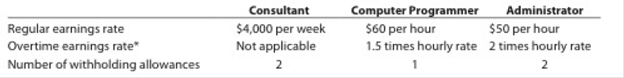Chapter 11, Problem 11.10EX

Chapter
Section
Textbook Problem

Calculate payrollBreakin Away Company has three employees—a consultant, a computer programmer, and an administrator. The following payroll information is available for each employee:*For hourly employees, overtime is paid for hours worked in excess of 40 hours per week.For the current pay period, the computer programmer worked 50 hours and the administrator worked 48 hours. 'The federal income tax withheld for all three employees, who are single, can he determined from the wage bracket withholding table in Exhibit 2 in the chapter. Assume further that the social security tax rate was 6.0%, the Medicare tax rate was 1.5%, and one withholding allowance is $75.Determine the gross pay and the net pay for each of the three employees for the current pay period. To determine Gross pay: The total amount such as salary, wages, commissions, overtime, and bonus paid by the employer to an employee in each pay period is called as gross pay. Net pay: It is the remaining amount of gross pay after all deductions. To Determine: The gross pay and the net pay for each employees for the current pay period. Explanation Working notes: Calculate withholding social security tax for Consultant. Social security tax = 6% ×$4,000=$240 (1) Calculate withholding Medicare tax for Consultant. Medicare tax = 1.5% of gross salary=1.5%×$4,000=$60 (2) Calculate the amount of federal income tax withheld for Consultant. Step 1: Calculate the amount subject to withholding. Amount subject to withholding = Gross earnings Withholding allowances amount=$4,000  ($75×2allowances)=$4,000$150=$3,850

Step 2: Calculate federal income tax withheld.

Federal income tax withheld = $890.02 + (33%×($3,850$3,700))=$939.52 (3)

Calculate regular earnings for Computer Programmer.

Regular pay = 40 hours ×$60 per hour=$2,400 (4)

Calculate overtime pay for Computer Programmer.

Overtime pay = (50 hours– 40 hours)×($60×1.5)= 10 hours×$90 per hour= $900 (5) Calculate withholding social security tax for Computer Programmer. Social security tax = 6% ×$3,300=$198 (6) Calculate withholding Medicare tax for Computer Programmer. Medicare tax = 1.5% of gross salary=1.5%×$3,300=$49.50 (7) Calculate the amount of federal income tax withheld for Computer Programmer. Step 1: Calculate the amount subject to withholding. Amount subject to withholding = Gross earnings Withholding allowances amount=$3,300  ($75×1allowances)=$3,300$75=$3,225

Step 2: Calculate federal income tax withheld

Still sussing out bartleby?

Check out a sample textbook solution.

See a sample solution

The Solution to Your Study Problems

Bartleby provides explanations to thousands of textbook problems written by our experts, many with advanced degrees!

Get Started